# you can choose more than one answer! Question 2 Suppose you have to use spherical coordinates...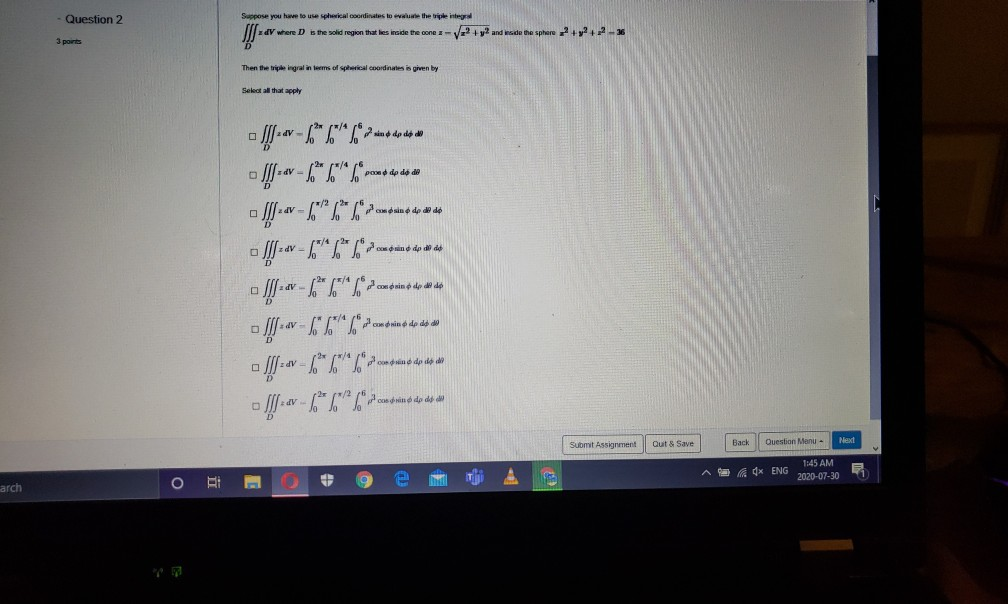you can choose more than one answer!

Question 2 Suppose you have to use spherical coordinates to the triple integy where is the solid region that lies incide the cone - V2 + y2 and wade the sphere 2+2+2-36 3 ports Then the triple ingral in terms of spical coordinates is given by Select all that apply *****,)..1-1 *****0,7.1.1-x-fift ******).T/9---fff *******<,)1,9---ff- - A---6"L" /dcbi* * * av - "L" /can din é do dys - [--- 6"""" povremo to use - ff --- 6*2/2 Submit Assignment Quit & Save Back Question Manu - Med 1:45 AN 4x ENG 2020-07-30 Bi arch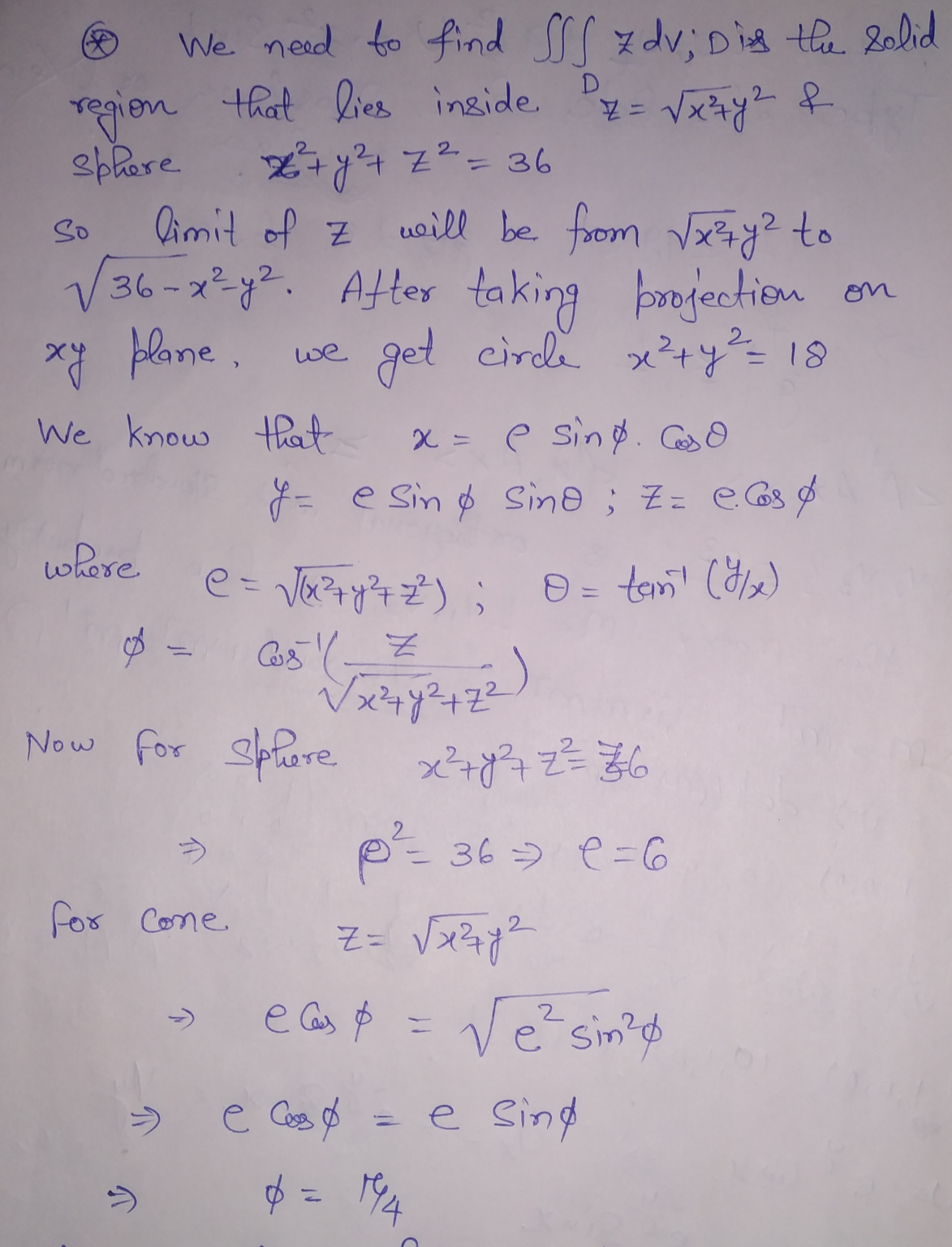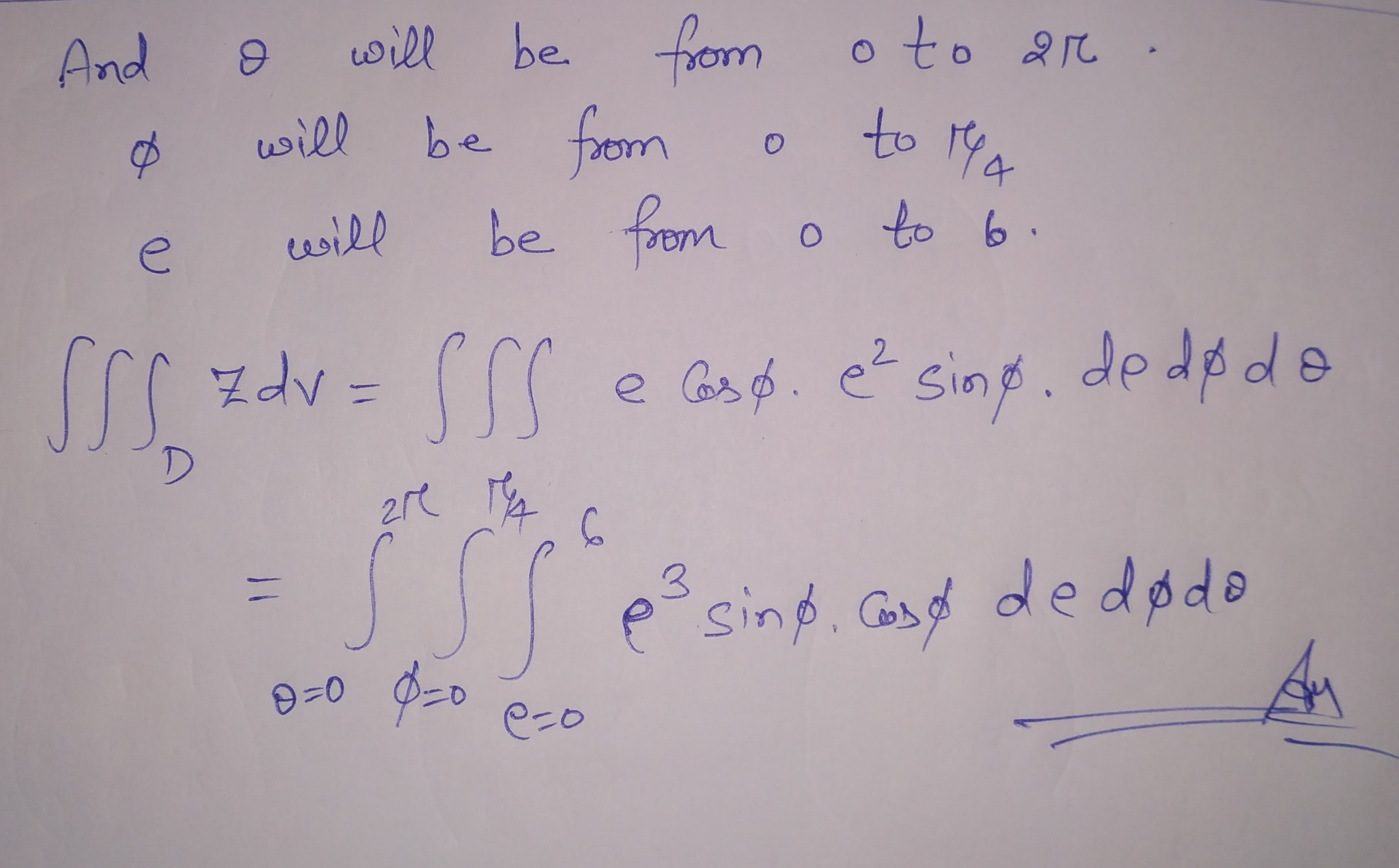##### Add Answer of: you can choose more than one answer! Question 2 Suppose you have to use spherical coordinates...
Similar Homework Help Questions
• ### Suppose you have to use spherical coordinates to evaluate the triple integral III z av where...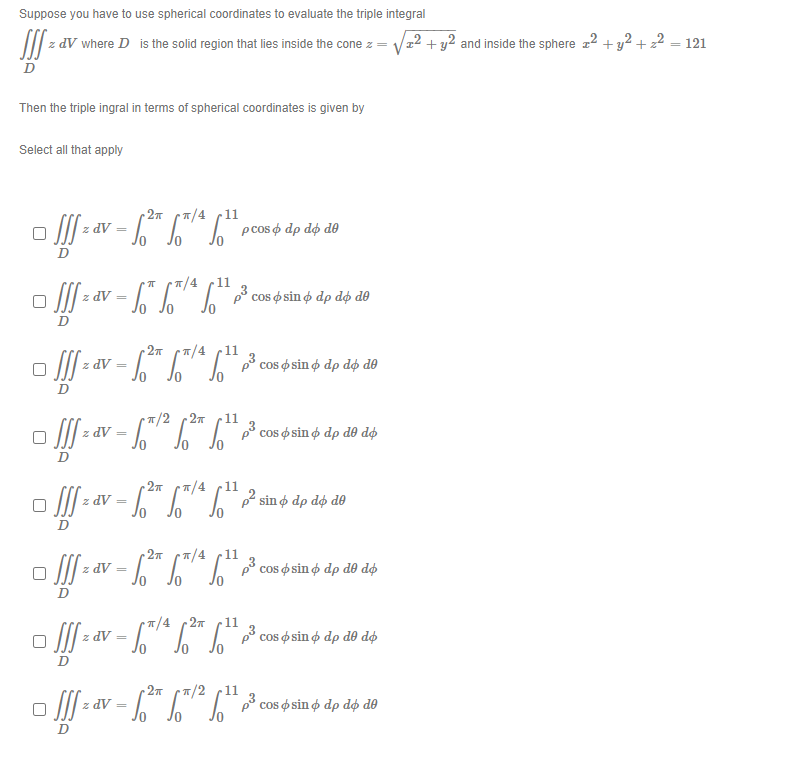Suppose you have to use spherical coordinates to evaluate the triple integral III z av where D is the solid region that lies inside the cone z = /22 + y2 and inside the sphere 22 + y2 + 2 = 121 D Then the triple ingral in terms of spherical coordinates is given by Select all that apply pcos o dp do de z dV = cos sin o dp do de D z DV = D pocos o...

• ### Suppose you have to use spherical coordinates to evaluate the triple integral SI z dV where...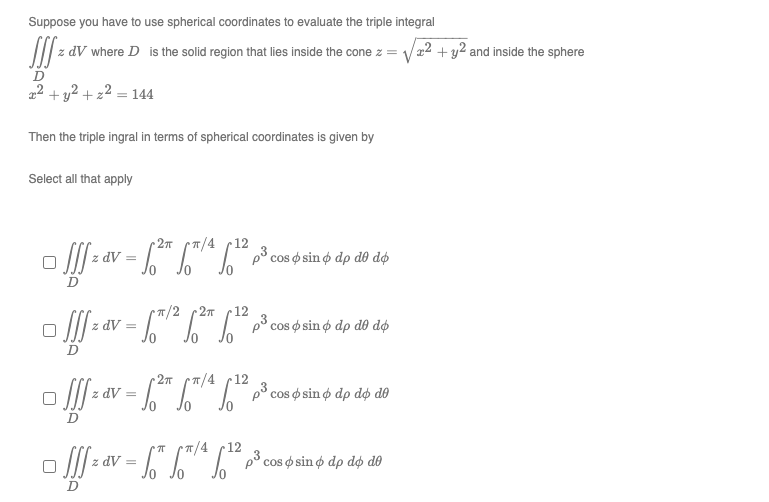Suppose you have to use spherical coordinates to evaluate the triple integral SI z dV where D is the solid region that lies inside the cone z = 22 + y2 and inside the sphere 22 + y2 +22 = 144 D Then the triple ingral in terms of spherical coordinates is given by Select all that apply p3 cos • sin o dp do do D [!] > av = 6*6** ? [!] > av = 6"* )*S" So*%*%**...

Free Homework App